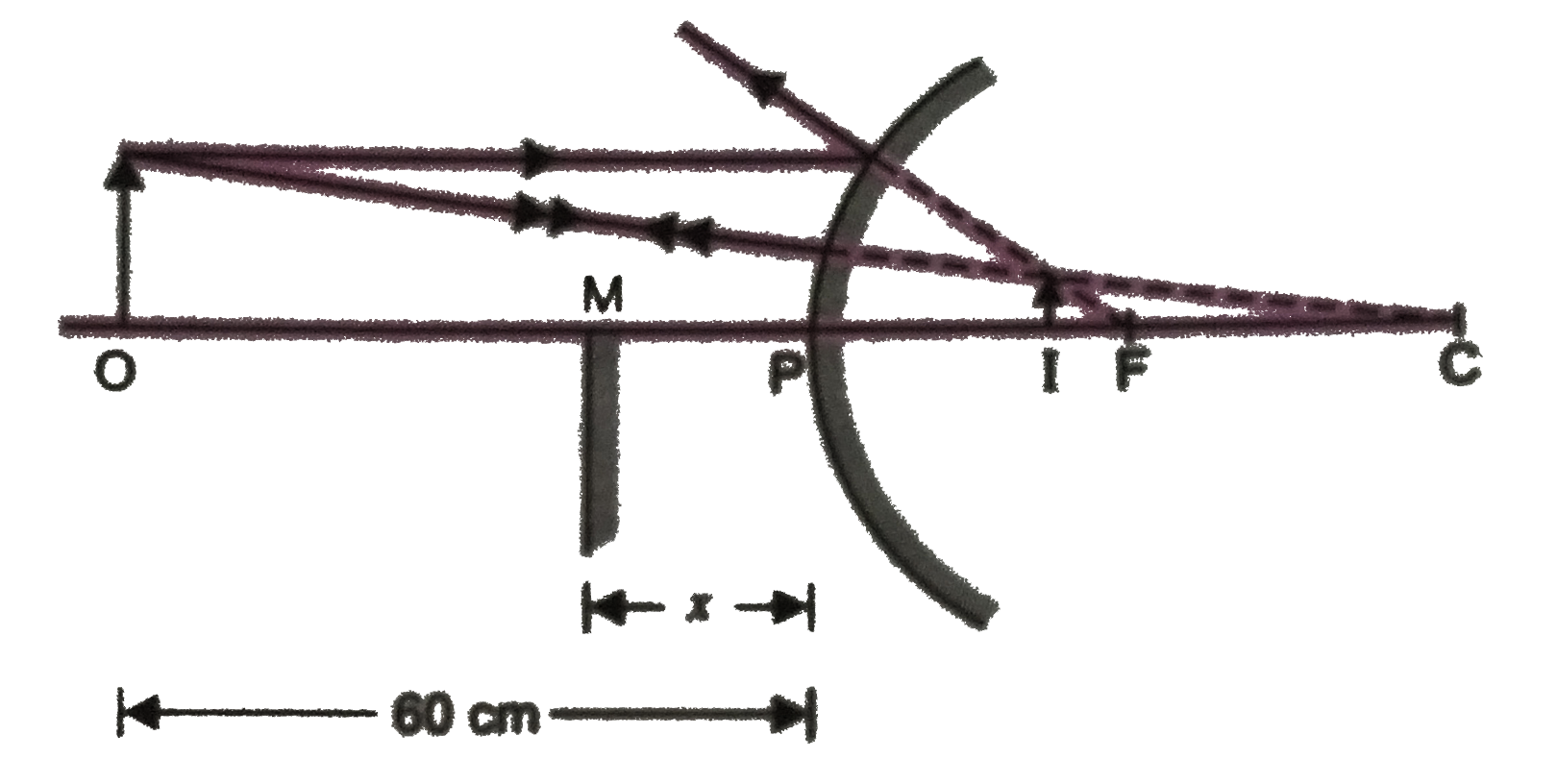# An object is held at a distance of 60 cm from a convex mirror of focal length 20 cm. At what distance from the convex mirror, should a plane mirror be

+1 vote
1.0k views
in Physics
closed
An object is held at a distance of 60 cm from a convex mirror of focal length 20 cm. At what distance from the convex mirror, should a plane mirror be held so that images in the two mirrors coincide?

+1 vote
by (71.8k points)
selected by

For convex mirror, u = -60 cm, f = +20 cm.From mirror formula, (1)/(v) =(1)/(f) - (1)/(u) = (1)/(20) + (1)/(60) = (3+1)/(60) = (1)/(15)
v = 15 cm. :. PI =15 cm.
Let the plane mirror be held at M so that images in the two mirrors coincide.
As OM = MI = MP +PI = x+15
and OM = (1)/(2)OI = (1)/(2)(60+15) = 37.5 cm.
:. x + 15 = 37.5, x = 37.5 -15 = 22.5 cm.